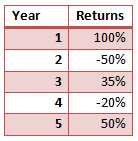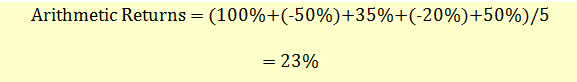# Time-weighted Returns

While calculating the returns on financial assets, we will often look at the returns from multiple holding periods. For example, one may hold an asset for five years, and the asset may have earned total 150% returns over this period of 5 years. However, it is difficult to interpret these returns as we cannot compare them with returns on other assets. For purpose of comparison, we will have to aggregate these returns for the same period such as daily returns, monthly returns, or yearly returns. It will be more suitable to calculate average annual returns than to know the returns earned over 5 years.While calculating the aggregate returns, our return measure will vary depending on what method we use to calculate the aggregate returns. Two common methods are arithmetic returns and geometric returns.

Let’s take an example to understand both these methods. Let’s say that our portfolio generated the following returns in 5 years.

Arithmetic Returns

To calculate the arithmetic average, we take the simple average of the 5 yearly returns as follows:Geometric Returns

One problem with arithmetic mean is that it assumes the returns on the investment made at the beginning of each period. So, for each period the beginning investment amount is assumed to be the same. It ignores the compounding effect of investment returns made in the previous years. Using arithmetic returns, our measure can be majorly flawed. Consider an investment of $100 at the beginning. Say in first year the investment value rises to$200. The returns are 100%. In year 2, the investment falls back to $100, which will be a return of -50% in the year 2. If we take the average of two year returns, i.e., 100% in year 1 and -50% in year 2, it shows an average annual return of 25% on this investment, even though our investment value is back to$100 (from where we started). This problem can be solved by calculating geometric returns which incorporates the compounding effect.